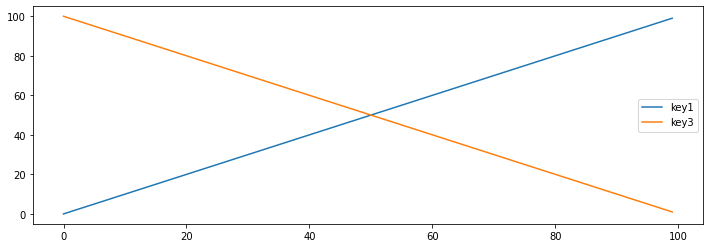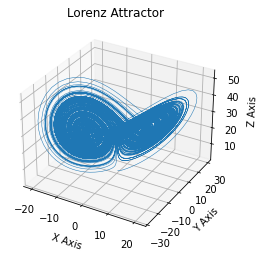# Streambook example¶

Streambook is a setup for writing live-updating notebooks in any editor that you might want to use (emacs, vi, notepad).

In [ ]:


In :
import numpy as np
import pandas as pd
import matplotlib.pyplot as plt
import time


## Main Code¶

Notebook cells are separated by spaces. Comment cells are rendered as markdown.

In :
x = np.array([10, 20, 30])


Cells that end with an explicit variables are printed.

In :
x

Out:
array([10, 20, 30])

Dictionaries are pretty-printed using streamlit and can be collapsed

In :
data = [dict(key1 = i, key2=f"{i}", key3=100 -i) for i in range(100)]


Pandas dataframe also show up in tables.

In :
df = pd.DataFrame(data)
df

Out:
key1 key2 key3
0 0 0 100
1 1 1 99
2 2 2 98
3 3 3 97
4 4 4 96
... ... ... ...
95 95 95 5
96 96 96 4
97 97 97 3
98 98 98 2
99 99 99 1

100 rows × 3 columns

In :
fig, axs = plt.subplots(figsize=(12, 4))
df.plot(ax=axs)
fig

Out:In [ ]:



By default, the notebook is rerun on save to ensure consistency.

In :
def simple_function(x):
return x + 10
y = simple_function(10)
y

Out:
20

Slower functions such as functions are loading data can be cached during development.

In :
def slow_function():
for i in range(10):
time.sleep(0.1)
return None
slow_function()


This uses streamlit caching behind the scenes. It will run if the arguments or the body of the function change.

## Longer example¶

In :
def lorenz(x, y, z, s=10, r=28, b=2.667):
"""
Given:
x, y, z: a point of interest in three dimensional space
s, r, b: parameters defining the lorenz attractor
Returns:
x_dot, y_dot, z_dot: values of the lorenz attractor's partial
derivatives at the point x, y, z
"""
x_dot = s*(y - x)
y_dot = r*x - y - x*z
z_dot = x*y - b*z
return x_dot, y_dot, z_dot

In :
dt = 0.01
num_steps = 20000

In :
def calc_curve(dt, num_steps):
# Need one more for the initial values
xs = np.empty(num_steps + 1)
ys = np.empty(num_steps + 1)
zs = np.empty(num_steps + 1)

# Set initial values
xs, ys, zs = (0., 1., 1.05)

# Step through "time", calculating the partial derivatives at the
# current point and using them to estimate the next point
for i in range(num_steps):
x_dot, y_dot, z_dot = lorenz(xs[i], ys[i], zs[i])
xs[i + 1] = xs[i] + (x_dot * dt)
ys[i + 1] = ys[i] + (y_dot * dt)
zs[i + 1] = zs[i] + (z_dot * dt)
return xs, ys, zs
xs, ys, zs = calc_curve(dt, num_steps)

In :
# Plot
fig = plt.figure(figsize=(12, 4))
ax.plot(xs, ys, zs, lw=0.5)
ax.set_xlabel("X Axis")
ax.set_ylabel("Y Axis")
ax.set_zlabel("Z Axis")
ax.set_title("Lorenz Attractor")
fig

Out:## Exporting to Jupyter¶

The whole notebook can also be exported as a Jupyter notebook.

The command is:

jupytext --to notebook --execute example.notebook.py

Some commands are slightly different in streamlit that jupyter.

In :
# Jupyter command
from IPython.display import HTML
HTML('<img src="example.gif">')
# Streamlit command

Out: